Reconstruction of the 2016 Cley Hill formation 1.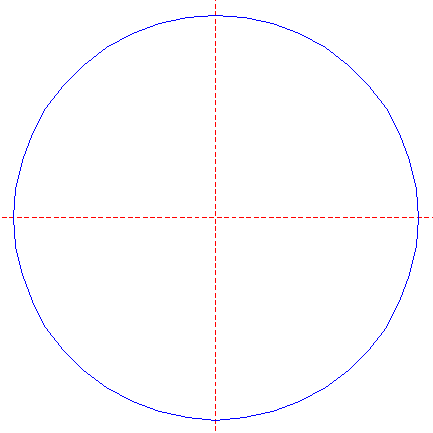Draw a circle. Draw and extend the horizontal and vertical centerlines. 2.Construct four inscribed pentagons (regular 5-sided polygons) of circle 1, one pointing up, one pointing down, one pointing to the left, and one pointing to the right. 3.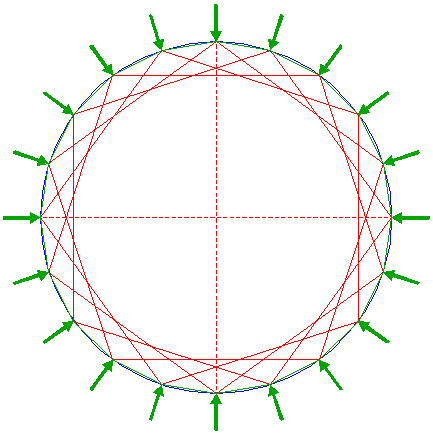Construct the inscribed regular 20-sided polygon of circle 1, by connecting all adjacent angular points of pentagons 2. 4.Draw the twenty rays of polygon 3, from the center to the angular points. Number these rays as well as the angular points 1 - 20 clockwise, starting at the top. 5.Construct a "two-points" circle (defined by the two end-points of a centerline) between angular points 3 nrs. 1 and 2. 6.Copy circle 5 to its lefthand intersection with circle 1. 7.Construct a circle concentric to circle 1, tangent to circle 6 at the upper side. 8.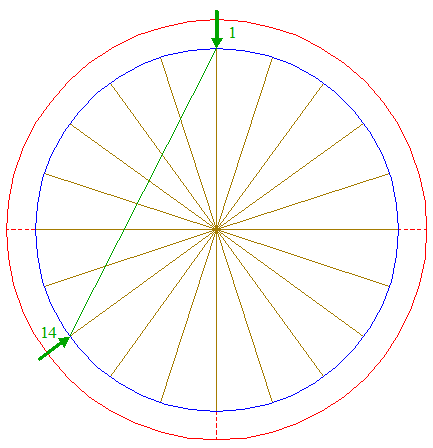Draw the connecting line between angular points 3 nrs. 1 and 14. 9.Construct a circle concentric to circle 1, passing through the intersection of line 8 and ray 4 nr. 20. 10.Construct the circumscribed decagon (regular 10-sided polygon) of circle 9, pointing to the right. 11.Construct the circumscribed circle of decagon 10. 12.Construct the circumscribed regular 20-sided polygon of circle 11, with the mid-point of the upper side on the vertical centerline. 13.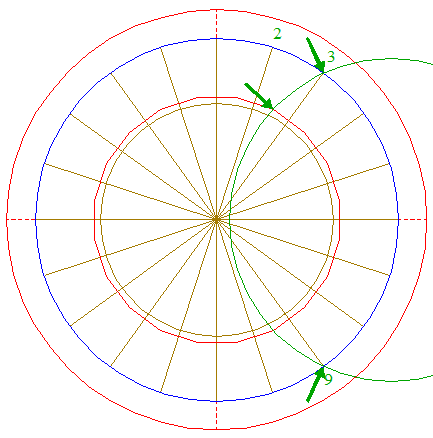Construct a circle passing through angular points 3 nrs. 3 and 9, and passing through the angular point of polygon 12 in between rays 4 nrs. 2 and 3. 14.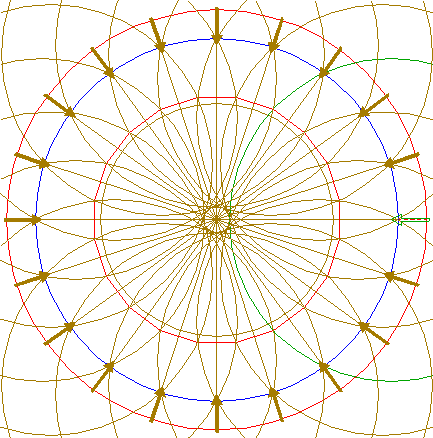Copy circle 13 nineteen times, by repeating step 13 for the corresponding angular points with respect to the other rays 4, as shown. 15.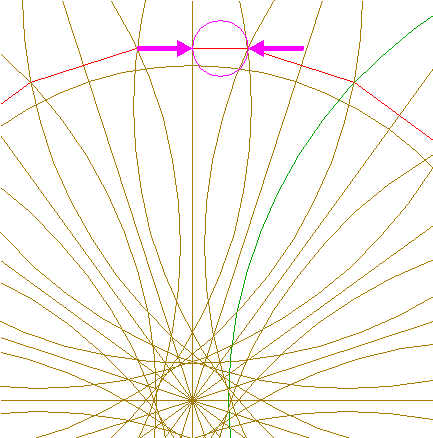Construct a "two-points" circle between the mid-point of the upper side of polygon 12 and its adjacent angular point to the right. 16.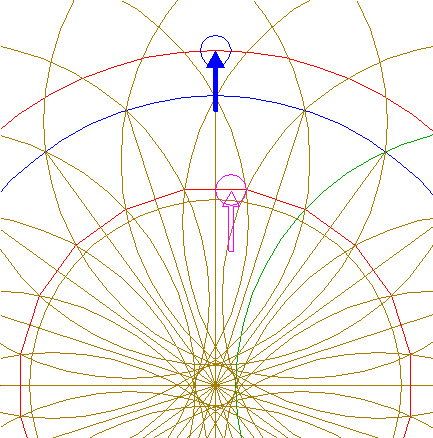Copy circle 15 to the upper intersection of circle 7 and the vertical centerline. 17.Construct a circle concentric to circle 1, tangent to circle 16 at the lower side. 18.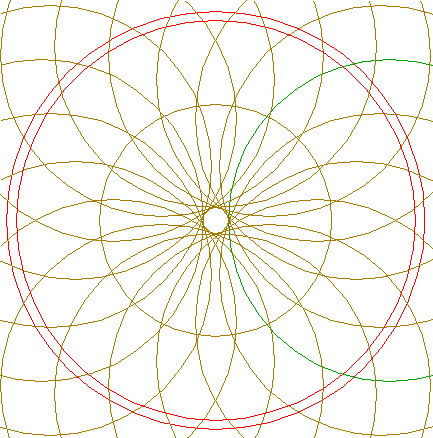Circles 7, 9, 13, 14, and 17, are used for the final reconstruction. 19.Remove all parts not visible within the formation itself. 20.Colour all areas corresponding to standing... 21....or to flattened crop, and finish the reconstruction of the 2016 Cley Hill formation. 22.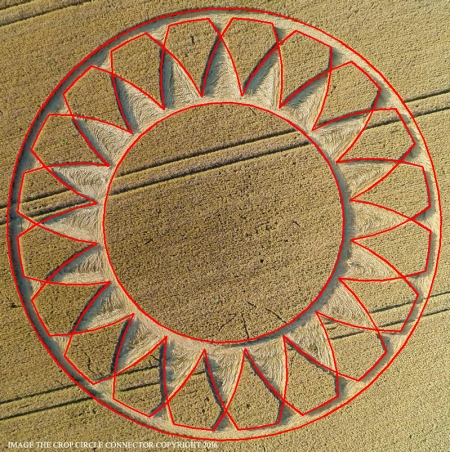The final result, matched with the aerial image.